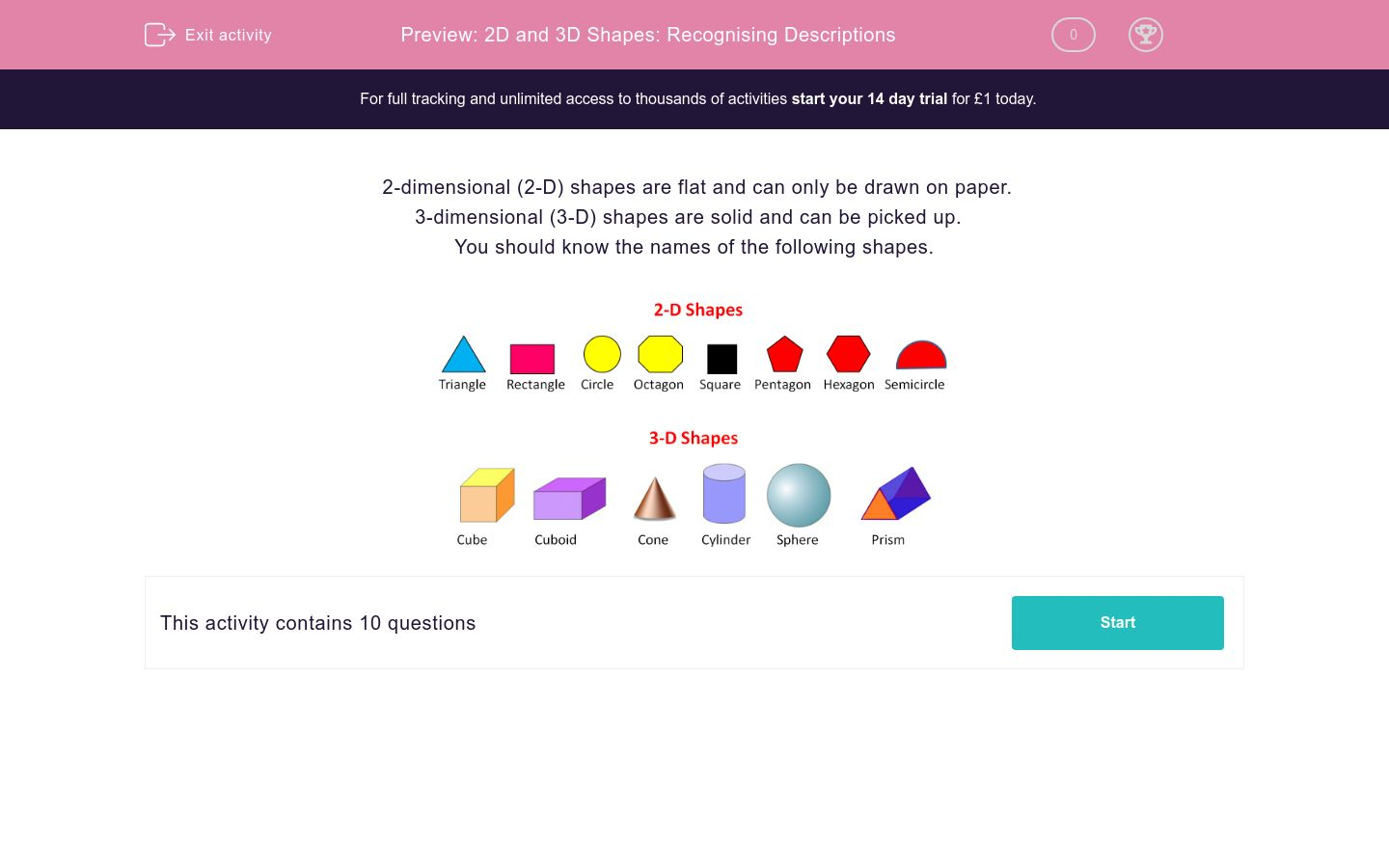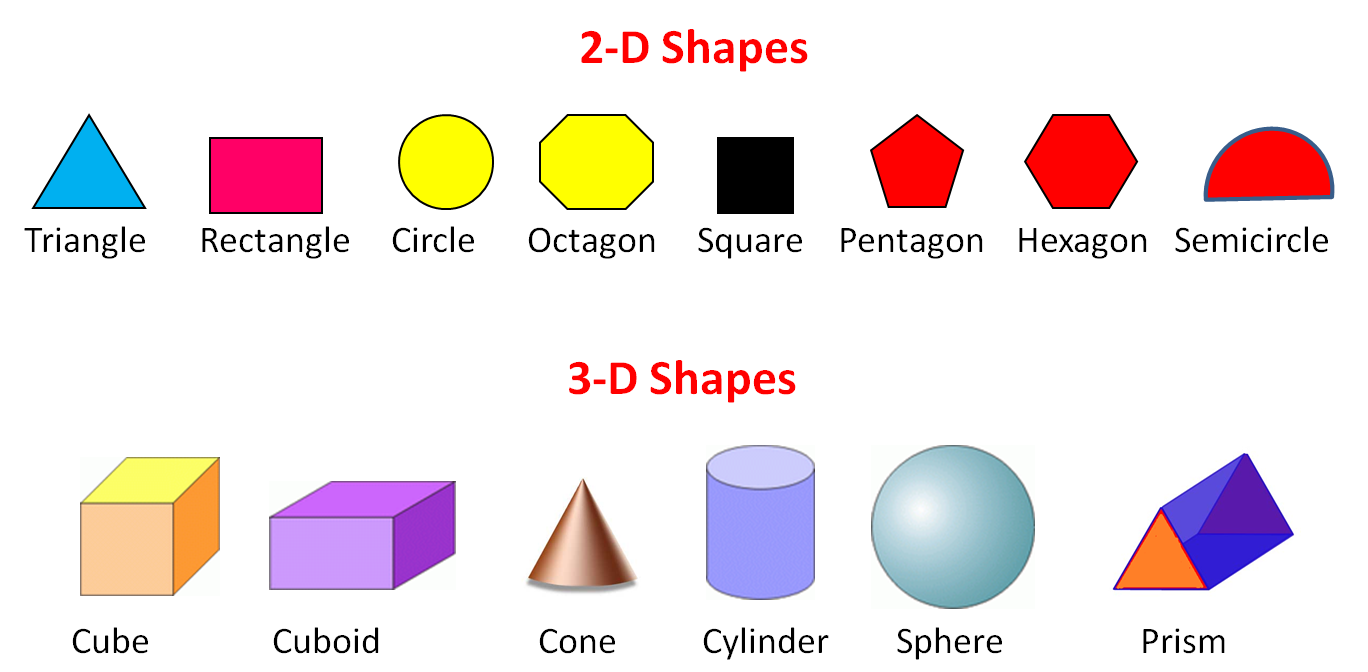# 2D and 3D Shapes: Recognising Descriptions

In this worksheet, students name the 2D and 3D shapes from the given clues.Key stage:  KS 2

Curriculum topic:   Maths and Numerical Reasoning

Curriculum subtopic:   3D Shapes

Difficulty level:### QUESTION 1 of 10

2-dimensional (2-D) shapes are flat and can only be drawn on paper.

3-dimensional (3-D) shapes are solid and can be picked up.

You should know the names of the following shapes.Name the following shape:

2-d with six sides

Pentagon

Hexagon

Cube

Name the following shape:

3-d with six faces, which are not all the same

Cuboid

Hexagon

Cube

Name the following shape:

3-d with six identical faces

Pentagon

Hexagon

Cube

Name the following shape:

2-d with five sides

Pentagon

Hexagon

Cube

Name the following shape:

3-d and round

Circle

Pyramid

Sphere

Name the following shape:

2-d and round

Circle

Pyramid

Sphere

Name the following shape:

Any 2-d shape with straight sides

Rectangle

Polygon

Name the following shape:

A 2-d shape with 8 corners

Pentagon

Polygon

Octagon

Name the following shape:

A 3-d shape with 8 corners

Cuboid

Cone

Octagon

Name the following shape:

A 2-d shape which is half a circle

Hemicircle

Demicircle

Semicircle

• Question 1

Name the following shape:

2-d with six sides

Hexagon
• Question 2

Name the following shape:

3-d with six faces, which are not all the same

Cuboid
• Question 3

Name the following shape:

3-d with six identical faces

Cube
• Question 4

Name the following shape:

2-d with five sides

Pentagon
• Question 5

Name the following shape:

3-d and round

Sphere
• Question 6

Name the following shape:

2-d and round

Circle
• Question 7

Name the following shape:

Any 2-d shape with straight sides

Polygon
• Question 8

Name the following shape:

A 2-d shape with 8 corners

Octagon
• Question 9

Name the following shape:

A 3-d shape with 8 corners

Cuboid
• Question 10

Name the following shape:

A 2-d shape which is half a circle